﻿ With respect to Nobu Shishido. KT88-based Push-Pull Amplifier

## With respect to Nobu Shishido. KT88-based Push-Pull Amplifier.

The scheme of a push-pull amplifier (the input stage and driver which made ​​based on this scheme, developed by genius of sound - Nobu Shishido (WAVAC audio lab)), was developed by famous tube designer - Michael Bron, ideas and sensible comments which helped me to implement this project, for which I am very thankful to him!

## Schematic of the Amplifier

The Amplifier consists of two modules: the module of Amplifier is shown in Fig. 1 and the module of Power Supply is shown in Fig. 2.

### Schematic of the Amplifier ModuleFig. 1. Schematic of the Amplifier Module

### Schematic of the Power Supply Module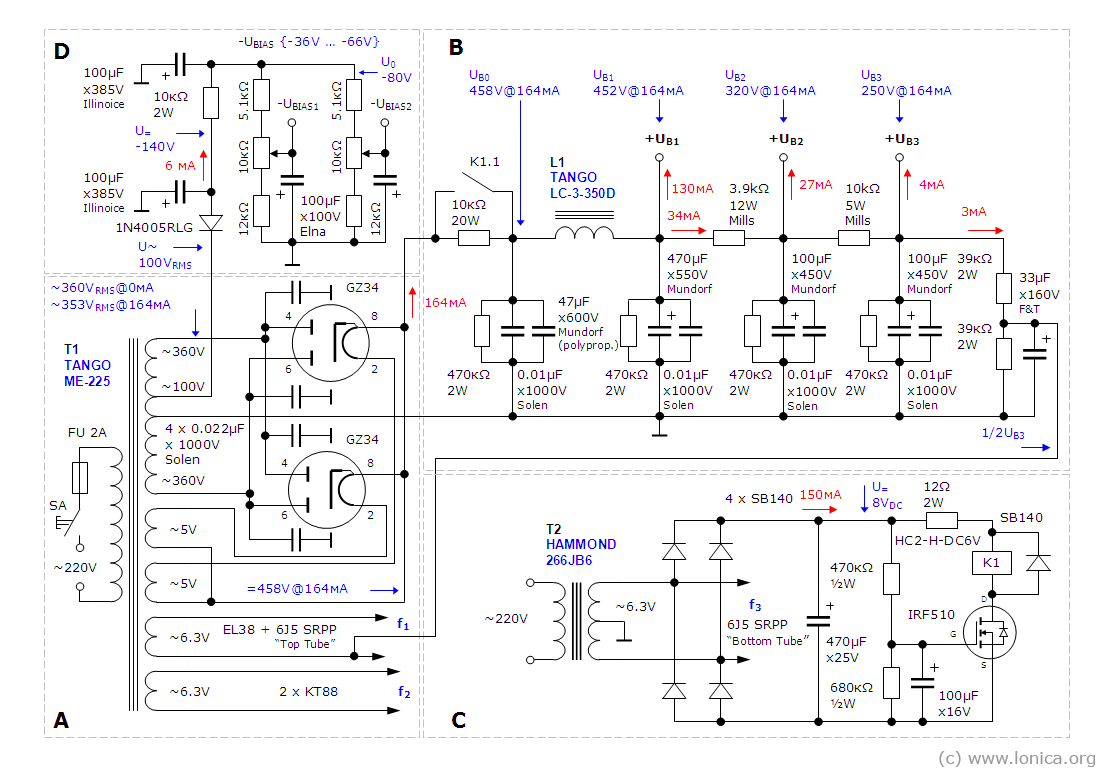Fig. 2. Schematic of the Power Supply Module

## Design of the Module of Power Supply

### Design of the Anode Rectifier (Section "A")

The basis of this module - power transformer МЕ–225 manufacturd by ISO Tango.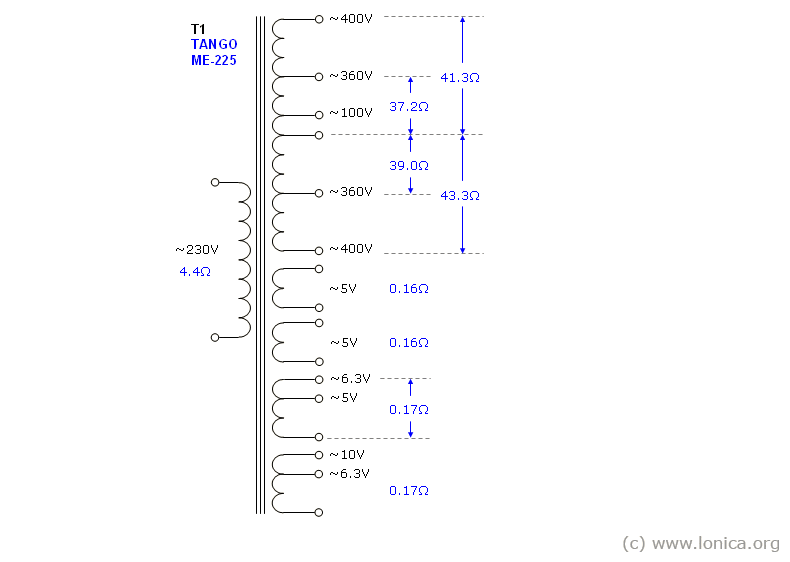Fig. 3. Power Transformer МЕ–225

with the following parameters:

Primary winding voltage (RMS) U1AC = 230V
Secondary winding voltages (RMS) U2AC = 400V–360V–0–100V–360V–400V (for power supply the anode circuits the taps 360V is used).
The rated current of the anode winding through the 400V tap - I2AC = 0.225A.

The power consumption of the transformer (calculated based on the secondary windings):

P2 = 2 x 5.0V х 3.3A + 6.3V х 3.3A + 10V x 3.3A + 400V x 0.225A = 177VA

Calculation of power consumption of the anode and filament circuits

Anode Winding

Resting Current of the output tubes: 2 х 65mА = 130mА
Resting Current of the driver tube: 27mА
Resting Current of the input tubes: 3.8mА
Current of the bias of filament of the "top" tube of the input stage: 2.5mА

Total Resting Current in the Anode Winding: 130 + 27 + 3.8 + 2.5 = 163.3mА (164mA).

Voltage on the Anode Winding: U2AC = 360V

Power Consumption of the Anode Winding: I2AC х U2AC = 0.164A х 360V = 59VA.

Filament Windings

Filament Current of the GZ34 Rectifier: 1.9A (both – 3.8А)
Filament Current of the КТ88: 1.6А (both – 3.2А)
Filament Current of the EL38: 1.4А
Filament Current of the 6J5G: 0.3А (the calculation takes into account only "top" tube of the SRPP stage, because "bottom" tube filaments is powered by a separate transformer)

Total Filament Current: 3.8А + 3.2А + 1.4А + 0.3А = 8.7А.

Power Consumption of the Filament Winding: 5.0V х 3.8А + 6.3V x 3.2А + 6.3V x (1.4А + 0.3А) = 19 + 20.6 + 10.7 = 50.3VA.

Total Power Consumption of the Secondary Windings: Р2 = 59VA + 50.3VA = 109.3VA.

The Power Trancformer Connections

Filament Windings of 0–5V 3.3А connected in parallel for supply the filament of both rectifier tubes.

Filament Winding of 0–5.0V–6.3V 3.3A with 6.3V tape is used for supply the filament of the "top" triode of the input stage and for driver tube. One of the outputs of this winding is connected to the voltage divider, so that half of the anode voltage of the input stage (DC bias) raises the potential of filament with a view to remove the potential difference between the cathode and the filament.

Filament Winding 0–6.3V–10.0V 3.3A with 6.3V tape is used supply the filament of the output (Push-Pull) stage.

Due to the DC bias is not supplied to the filament of the "bottom" tube of the SRPP, then the filament of the "bottom" tube, as well as anode voltage delay circuit is powered from a separate filament transformer T2 - 266JB6, manufactured by Hammond.

Measured resistance of one half of the anode winding of the transformer = 41.3Ω (400V tape) and 37.2Ω (360V tape), the second half of the anode winding – 43.3Ω (400V tape) and 39Ω (360V tape), so the average value of resistance of the half of anode winding of the transformer RТР2 = 42.3Ω (400V tape) или 38.1Ω (360V tape) can be taken for further calculations.

The transformation ratio (ratio of the number of turns of the primary winding to secondary windings or ratio of the voltage on the primary winding to the secondary winding) of the anode winding (360V tape):

nР = UА / U2АС = 230V / 360V = 0.64.

The measured resistance of the primary winding of the transformer RТР1 = 4.4Ω.

Resistance of the transformer from the secondary (anode) winding: RТР = RТР2 + RТР1 / nР = 48.8Ω.

### Static Load operation mode of the Anode Rectifier

If there is no input audio signal (idle mode), the amplifier is the static load for the rectifier with total current of anode circuit IР = 164mА and total current of filament circuit IF = 8.7А.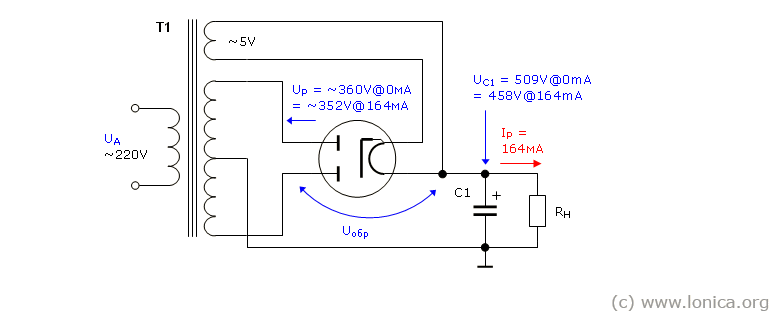Fig. 4. Circuit of the rectifier operating on the static load

Voltage drop on the anode winding of the transformer

Anode circuit current IР = 164mА, flowing through the anode winding of the transformer with active resistance 48.8Ω, lead to voltage drop across this winding: 0.164А х 48.8Ω = 8V. Therefore, the voltage UР, supplied to the anodes of the rectifier, will be: U2АС – 8V = 352V.

Voltage drop on the rectifier.

Because two rectifiers are connected in parallel, through an one diode will flow only half the current, i.e. 164 mА / 2 = 82mА. For GZ34 tube (see. Fig 5) for current 0.082А voltage drop on one diode is 13.5V.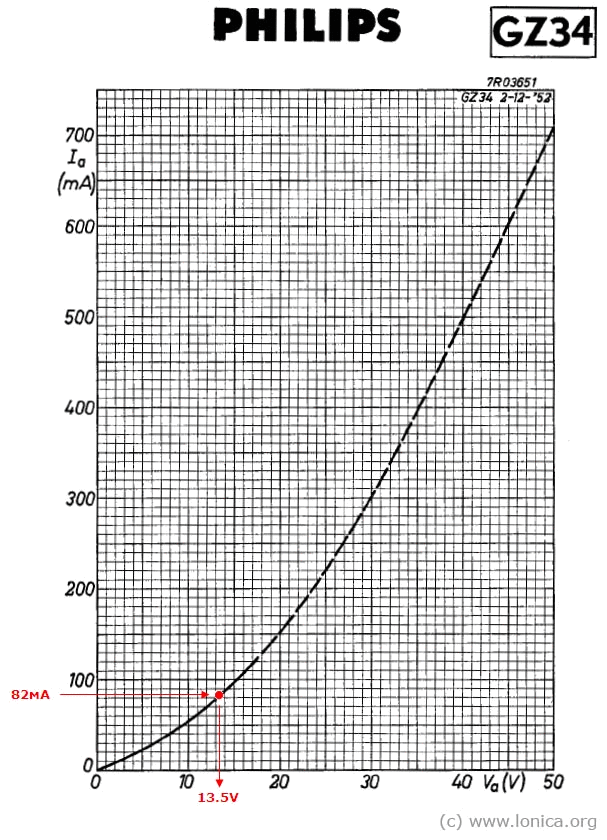Fig. 5. Plate Characteristics GZ34 (by Philips Data Handbook) from frank.pocnet site)

Thus the total voltage drop on the active resistance of the anode winding of the transformer and rectifiers is: ΔU = 8V + 13.5V = 21.5V.

Direct voltage applied to the anodes of the rectifier in idle mode: UP0 = √2 х U2AC = √2 х 360V = 509V. A first capacitor of the filter in idle mode is charged prior to this voltage.

Operating voltage of the first capacitor of the filter should be about 10% more than this voltage, i.e. 509 + (509 х 0.1) = 560V (600V).

Since the anode winding and the first capacitor of the filter are connected to the rectifier in series, then when the negative half cycle of the voltage applied to the anode (rectifier is closed) the cathode of the rectifier is under a positive voltage of the first capacitor of the filter. Thus, a voltage between the anode and the cathode of the closed rectifier is double of the amplitude of the anode winding (Peak Inverse Voltage): UPEAK = 2 х UP0 = 2 х 509 = 1018V.

Voltage on the cathode of the rectifier.

UК = √2 x (U2AC – ΔU) = √2 x (360V – 21.5V) = 479V.

The amplitude of the voltage ripple across the capacitor С1 (47μF):

UC1~ = IOUT / (2 x fC x C) = 0.164 / (2 x 50 x 47e–6) = 35V (p–p).

DC voltage on the capacitor С1: UС1 = UК – UC1~/2 = 479 – 35/2 = 461V.

The load of the rectifier can be assumed as: RН = UOUT / IOUT = 461 / 0.164 = 2811Ω. (with taking into account the 40Ω resistance of the choke, the resistance of the rectifier load becomes equal to 2851Ω).

### Design of the Choke Filter (Section "B")

To further reduce of the ripple of the voltage, the choke filter (see. Fig. 6) is used. The filter is based on the choke LC–3–350D manufacturd by ISO Tango. with following parameters:

L = 3H.
IRATED = 350мА
IMAX = 450мА
R = 40ΩFig. 6. The Choke Filter

Since the choke has an active resistance, the voltage at the filter output (UC2) is less than the input voltage (UС1) by the IР х 40Ω. For 164mА load the voltage drop becomes equal to 6.6V, so the voltage on the capacitor С2 at 164mА load will be 454.4V.

Filtration factor of the circuit КF = 4 х π2 х f2 x L x C2, where

f – frequency of pulsation of the filtered voltage (for a full-wave rectifier circuit the frequency of pulsation is 100Hz).
L – inductance of the choke, H.
С – capacity of the capacipor, following the choke (С2), F.
shows by how many times the ripple voltage at the output of the filter is less the ripple voltage at the input of the filter, i.e. КF = UC1~ / UC2~.

Thus, for the capacitor С2 = 470μF, the КF= 4 х π2 х 1002 x 3 x 470e–6 = 556.6 and ripple voltage at the output of the filter UC2~ = UC1~ / КF = 35 / 556.6 = 0.063Vp–p.

The operating voltage of the capacitor at the output of the choke in accordance of the slight ripple voltage may be selected by approximately 5% greater than the output voltage of the filter, i.e. = 454.4V + 0.05 х 454.4V = 477V (it is possible to use a capacitor with a standard operating voltage - 550V).

Further filtering of ripple can be achieved by using the rejector, consisting of a choke L1 and capacitor C3, connected by parallel to the choke (parallel resonance circuit). The circuit has a maximum resistance on the 100 Hz resonant frequency. The circuit can be calculated on the basis of the following rule.

The condition of the resonance of currents: YC = YL (where Y is conductivity), so ωC = 1/ωL, so ω = 1/√(LC). Given, that ω = 2πf, the f (100 Hz) = 1/(2π√(LC)). For the 3 H choke, the shunt capacitor value will be equal: CS = 1/(L x (2 x π x f)2) = 1/(3 x ((2π x 100)2)) = 0.844μF (a standard value is 0.82μF).

The minimum value of the current flowing through the Choke: IMIN = 2 x √2 x UC2 / (6 x π2 x f x L) = 2 x √2 x 461V / (6 х π2 х 100 x 3) = 73mА. when value of the load current is less than this minimum value, then the smoothing capacitor, connected after the choke, will be charged to the peak value of the voltage at the cathode of the rectifier under load, i.e.. up to 479V.

### Calculation of anode resistors for the stages of the amplifier (Section "B")

Estimated value of the anode voltage of the output stage: UB1 = 452V at IB1 = 130mА.

Estimated value of the anode voltage of driver stage UB2 = 320V at IB3 = 27mА thus, the value of the driver tube anode resistor is equal to (UB1 – UB2) / (27mА + 4mА + 3mА) = 3.9кΩ.
Power dissipation of this resistor: (UB1 – UB2) х (27mА + 4mА + 3mА) = 4.5W

Estimated value of the anode voltage of the input stage UB3 = 250V at IB3 = 4mА thus, the value of the input stage anode resistor is equal to (UB2 – UB3) / (4mА + 3mА) = 10кΩ.
Power dissipation of this resistor (UB2 – UB3) х (4mА + 3mА) = 0.5W

A current, flowing through a DC bias voltage divider is: I = 3мА, thus, the value of the resistance of this divider is equal to UB3 / 3mА = 83кΩ.

### Calculation of the delay circuit of the anode voltage (Section "С")

Response time of the circuit τ = C x (R1 x R2 / (R1 + R2)).

So, with the values С = 100μF, R1 = 470кΩ, R2 = 680кΩ, the τ is 28 seconds.

### Design of the grid fixed bias rectifier (Section "D")

The grid bias range is: UBIAS = {–35 ... –70}V, i.e. voltage drop across the regulating resistor will be 30V.

AC input voltage of the rectifier: U~ = 100V.

DC output of the rectifier: U= = √2 х 100V – U drop on diode = 141V – 1.0V = 140V.

The DC Filter resistor: RF = 10кΩ.

Common current of two voltage dividers: I0 = 6mА, so voltage drop on the DC Filter resistor: UR = 10кΩ x 6mА = 60V.

Thus, the voltage applied to the two dividers: U0 = √2 x 100V – Udiode – UR = 141 – 1.0 – 60 = 80V, and resistance of one divider: R = U0 / (I0 / 2) = 80V / 3mА = 27кΩ.

A current, flowing through each divider: I1 = I2 = 6mА / 2 = 3mA.

A bottom resistor of the divider is chosen from the condition of the lower limit value of the bias voltage -35V: 35V / 3mА = 11.7кΩ (a standard value of 12кΩ is used, the lower limit value of the bias voltage will be –36V).

The potentiometer of the divider should provide a change in voltage from 36V to 70V, so the voltage drop across the potentiometer will be 70V - 36V = 34V, at a current of 3mA, the voltage drop determines its resistance equal to 34V / 3mA = 11.3kΩ. (a potentiometer of 10кΩ is used, and the range of grid bias voltage adjustment is 10кΩ х 3mА = 30V).

A top resistor of the divider is: 27кΩ – (12кΩ + 10кΩ) = 5кΩ (a standard value of 5.1кΩ is used).

The DC filter resistor RF power dissipation is: 10кΩ х 6mА2 = 0.36W.

## Design of the Output Stage of the Amplifier

Since the output stage utilized a ultralinear connection to the specified output transformer XE-60-5, manufactured by ISO Tango then the calculation reduces to determining the idle current and power dissipation of the stage.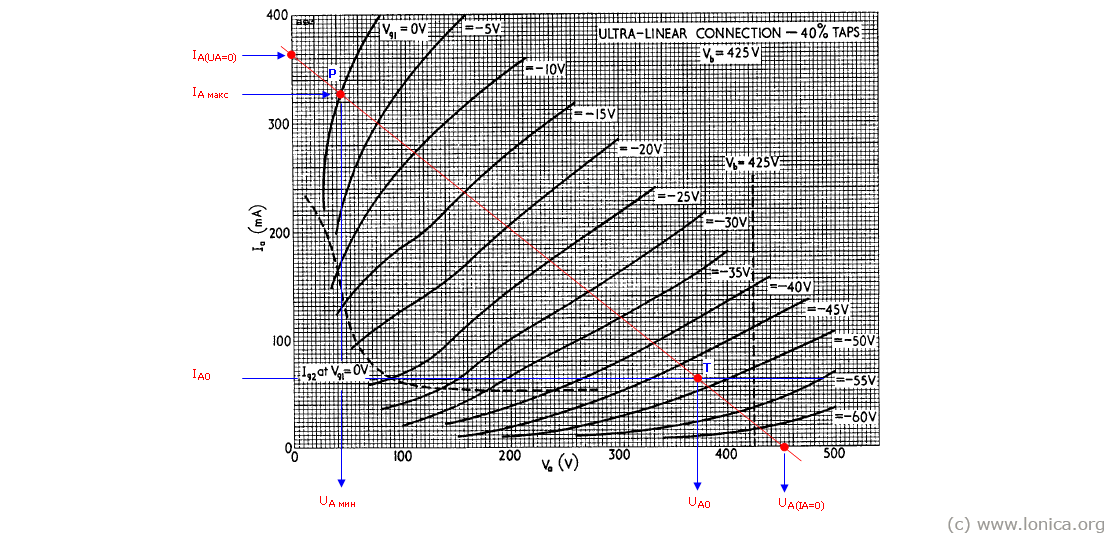Рис. 7. Operation mode of the KT88 tube in push-pull output stage (the tube datasheet (by The General Electric CO. LTD of England) can be downloaded from frank.pocnet site)

First point of the loadline IА (UА = 0) = EА / RА, где RА is determined based on the resistance RА–А of the output transformer XE–60–5 (5кΩ), recalculated for one tube: RА = RА–А / 4 = 1.250кΩ. Then IА (UА = 0) = 452 / 1.250 = 362mА.

Second point of the loadline UА(IА = 0) = EА = 452V.

The point "Р" is defined at the intersection of the loadline with the characteristic at UС = 0, wherein IА max = 328mA, UА min = 42V.

The idle current of the tube IА0 = ~(1/3 ... 1/5) IА max / 2 = 65mА (Point "Т") is located at the intersection of the loadline with the characteristic at UС approximately equal -43V, this is a voltage offset of the tube in idle mode.

Point "Т" determines a plate voltage in the idle mode: UА0 = 370V, relative to the idle current of the tube IА0.

Resistance in the anode circuit of two tubes: RА–А = 22 x (UА0 – UА min) / (IА max – IА0) = 4 x (370 – 42) / (0.328 – 0.065) = 5кΩ.

Power dissipation of the anode: PA = UА0 x IА0 < PA max = 370 х 0.065 = 24W < 40W.

The maximum power given to the load by two tubes with the efficiency of the ultralinear connection ~60%: P~ = (IА max x (UА0 – UА min) x η) / 2 = (0.328 x (370 – 42) x 0.60) / 2 = 32W.

The amplitude of the AC component of the anode current of the tube: I = (IА max – IА0) / 2 = (328 – 65) / 2 = 132mА.

Max. effective value of of the anode current of the tube at maximum power: IА0 max = (IА max + 2 x IА0) / 4 = (328 + 2 x 65) / 4 = 115mА.

Max effective value of of the anode current flowing through output transformer Imax = 2 х IА0 max = 230mА.Fig. 8. Creation of IА (UG) characteristics of tube KT88 of the Push-Pull output stage (the tube datasheet (by The General Electric CO. LTD of England) can be downloaded from frank.pocnet site)

The peculiarity of this output stage is the presence of feedback, which is provided from the output transformer to the cathodes of the output tubes (i.e. "super-triode" connection). Details on this scheme is described in "Universal system and output transformer for valve amplifiers" brochure, see Menno van der Veen site.

## Design of the Input Stage of the Amplifier

The input stage is created based on Shunt Regulated Push-Pull (SRPP) design.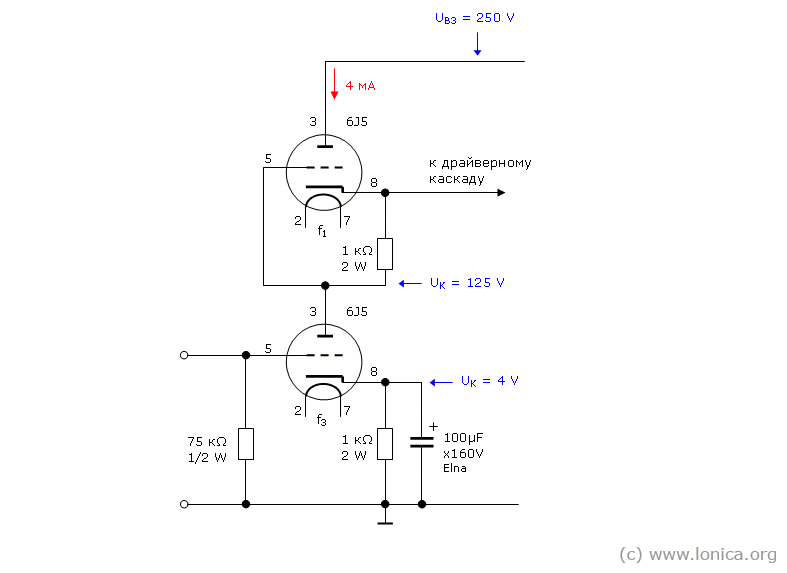Fig. 9. Schematic of the input stage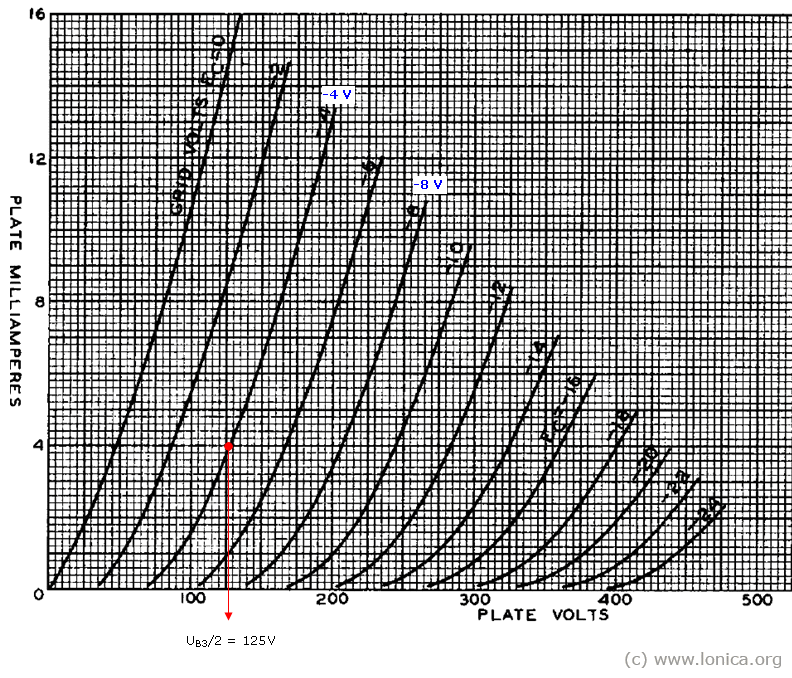Fig. 10. Plate characteristics of the 6J5G tube (the tube datasheet (by RCA) can be downloaded from frank.pocnet site)

For a given idle current 4mA, flowing through the bottom tube, the grid voltage of the tube will be 4V, so resistance of the self-bias in the cathode of the bottom tube (as well as of the top tube) will be 4V/4mA = 1kΩ.

Amplification factor of this stage, assuming that as a "top" and "bottom" are used identical tubes, and the cathode resistor of the "bottom" tube is shunted by a capacitor:

А = μ х (rА2 + RК2 х (μ + 1)) / (rА1 + rА2 + RК2 х (μ + 1)) = 20 х (8000 + 1000 х (20 + 1)) / (8000 + 8000 + 1000 х (20 + 1)) = 15.7.

Where:

rА1 – internal resistance of the "bottom" tube
rА2 – internal resistance of the "top" tube
RK2 – resistance of the self-bias of the "top" tube

μ – amplification factor (gain) of the tube

The amplifier is designed for a nominal input voltage of the audio signal ~1.0VP–P, so at this level of the input signal, the output voltage of this stage will be 1.0 х 15.7 = 15.7VP–P. Since the connection between the input stage and the driver stage is direct, so the value of the grid voltage of the driver tube will be: UК + 15.7/2 = 125+7.85 = 133V.

## Design of the Driver Stage of the Amplifier

As noted earlier, the voltage of bias UК of the driver tube (voltage drop on the cathode resistor) should be at least 133V. With selected current, flowing through anode of the tube А0 = 27mА, cathode resistor of the tube RК will be 133/27 = 5кΩ. Power dissipation of the cathode resistor P = UК х IА0 = 133V x 0.027mА = 3.6W.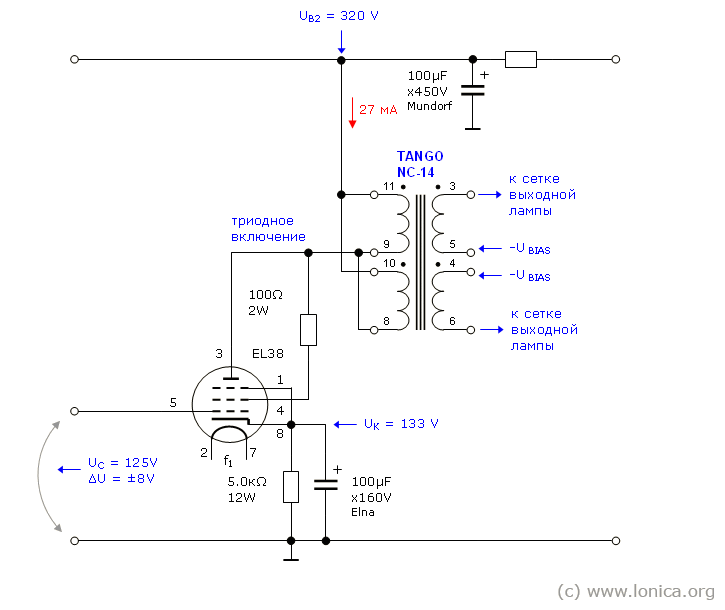Fig. 11. Schematic of the driver stage

As an interstage transformer, the NC–14 transformer, manufactured by ISO Tango has been selected. Full impedance of anode windings of the transformer (connected in parallel) is 1.25кΩ (resistance is 82.5Ω), admissible current is 30 mА. Full impedance of anode windings of the transformer (connected in series) is 5кΩ (resistance is 0.33кΩ), admissible current is 15 mА.Fig. 12. NC–14 Interstage Transformer

DC voltage on the grid of the tube in idle mode: U C0 is 125V, cathode resistor R K is 5kΩ (voltage of DC bias at the selected idle current I A0 = 27mA, U K = 133V), so on the grid of the tube there is a constant bias voltage relative to the cathode: U C = 125 - 133 =-8V (operation point of the tube).

Load line (see. Fig. 13) for DC current, divides the anode voltage between the tube (Ri) and resistors in the anode (RА) and cathode (RК) circuit, built on the basis of the following considerations:

1. If the anode current is zero, the voltage at the anode of the lamp is equal to power source voltage ЕА = 320 V.

2. If the voltage drop on the tube is equal to zero, then the current through the tube is limited to IА max = ЕА/(RА + RК). For a given RА = 0.0825кΩ (resistance of anode windings of the transformer connected in parallel) and RК = 5.0кΩ, the IАмакс will be 320 / (0.0825 + 5.0) = 63мA.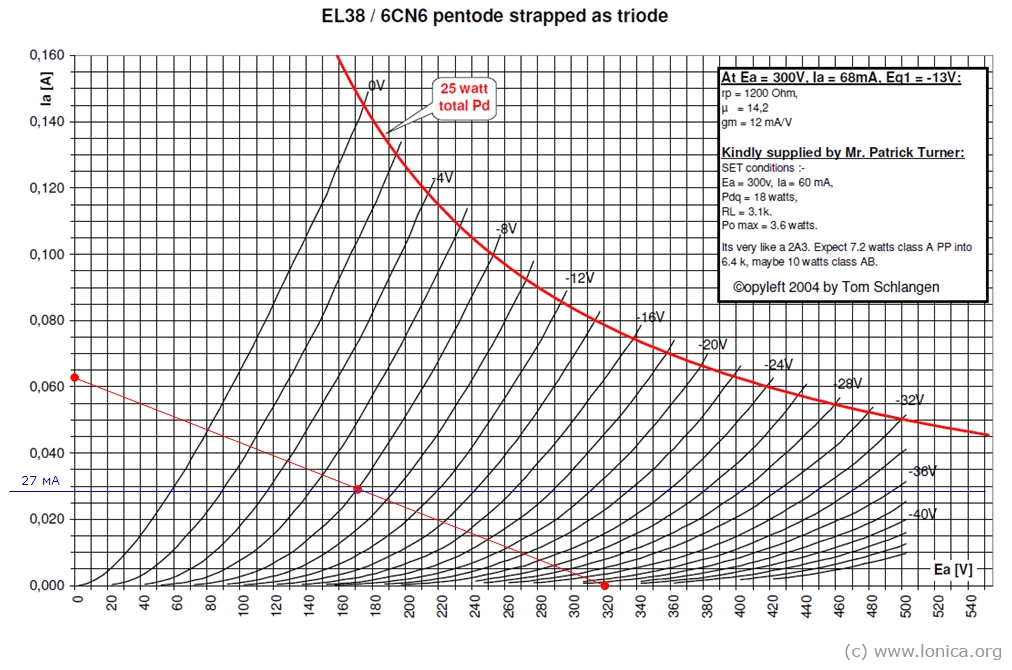Fig. 13. Plate Characteristics of the EL38 tube (triode connection) by Tom Schlangen

## Parts List of the Amplifier

### Mechanical Parts

 Chassis: Hammond Chassis Walnut P-HWCHAS1310AL 2 pcs. Hammond Bottom Panel P-HHW1310ALPL 2 pcs. Terminal Strip (a distance between lugs is 9.525 mm): 47.6 mm 6 Lug P-0602H 10 pcs. 57.2 mm 7 Lug P-0702H 10 pcs. 66.6 mm 8 Lug P-0802H 10 pcs. Assembly Pads MPSA 35 – 50 mm for MUNDORF M-Lytic AG Series Capacitors MUNDORF-75217 6 pcs. Bias regulator Knob P-K310 4 pcs. Tube Sockets (CNC) 14pcs. Spacer М4 30mm F-F 8 pcs. Spacer М4 10mm M-F 16 pcs. Spacer М3 10mm M-F 8 pcs. Spacer М3 10mm F-F 8 pcs. Screw М4 х 6mm 100 pcs. Screw, countersunk head М4 х 6mm 100 pcs. Screw М3 х 6mm 100 pcs. Screw, countersunk head М3 х 20mm 100 pcs. Lock Washer М4 100 pcs. Lock Washer М3 100 pcs. Washer М4 100 pcs. Washer М3 100 pcs. Nut М4 100 pcs. Nut М3 100 pcs. Aluminum Plate 2.3 mm 304 mm х 914 mm 1 pcs.

### Electromechanical Parts

 Wire insulated single core 21.5 AWG 1 reel Wire insulated single core 16.5 AWG 1 reel Teflon insulation inner ø 1.5mm outer ø 1.8mm 7.5m Terminals for connecting the speakers 12 pcs. RCA Connectors "D" (audio inputs) NF2D-B-0 2 pcs. Terminal of Anode Voltage (Pomona) 2142-0 2 pcs. Plug of Anode Voltage (Pomona) 3690-0 2 pcs. Plate Caps (Yamamoto) 6mm 320-070-91 2 pcs. Panel Meter (Yamamoto Precision Panel Meter) 100mА 320-059-18 2 pcs. Power Terminal (IEC) + Fuse 2 pcs. Power Switch (Nikkai) 2 pcs. Switch for measure the idle current of the output stage (Nikkai) 2 pcs.

### Electronic Parts

 Power Transformer (ISO Tango) МЕ–225 2 pcs. Filament Transformer (Hammond) 266JB6 2 pcs. Choke (ISO Tango) LC–3–350D 2 pcs. Interstage Transformer (ISO Tango) NC–14 2 pcs. Output Transformer (ISO Tango) XE–60–5 2 pcs. Rectifier GZ–34 4 pcs. Tube (GEC) 6J5GT 4 pcs. Tube (Mullard) EL38 2 pcs. Tube (Gold Lion) KT88 4 pcs. Electrolytic Capacitor, Mundorf, M-TubeCap 47μF х 600V 2 pcs. Electrolytic Capacitor, Mundorf, M-Lytic HV 470μF х 550V 2 pcs. Electrolytic Capacitor, Mundorf, M-Lytic MLSL HV 100μF + 100μF x 500V 2 pcs. Resistor, Mills, MRA–12 20кΩ 12W 4 pcs. Resistor, Mills, MRA–12 3.9кΩ 12W 2 pcs. Resistor, Mills, MRA–5 10кΩ 5W 2 pcs. Electrolytic Capacitor, Elna Silmic II 100uF 16V 2 pcs. Electrolytic Capacitor, Elna Silmic II 470 uF 25 V 2 pcs. Electrolytic Capacitor, Elna Silmic II 100uF 100V 4 pcs. Relays of the anode voltage delay (Panasonic) HC2-H-DC6V-F 2 pcs.

## Mechanical Circuits

### Parts of the Amplifier. Top View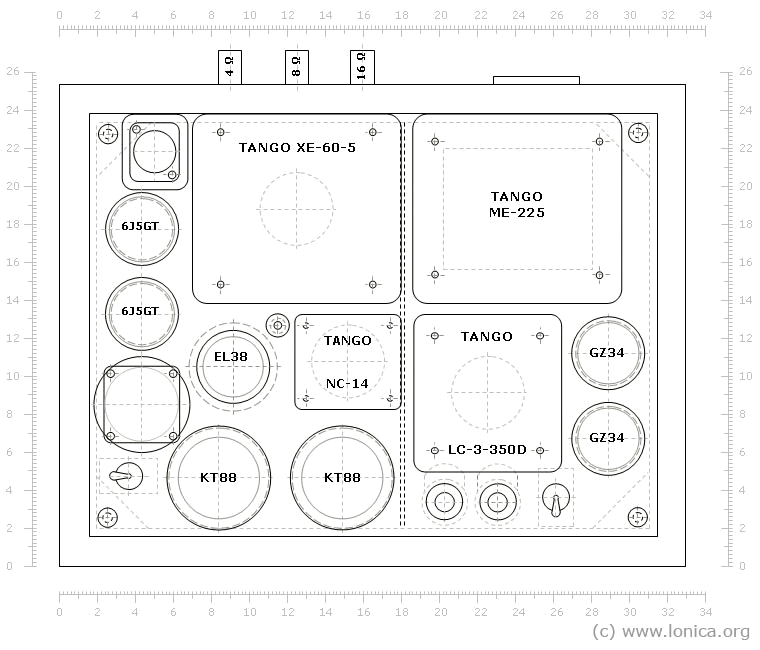Fig. 14. Parts of the Amplifier. Top View

### Parts of the Amplifier. Rear ViewFig. 15. Parts of the Amplifier. Rear View

### The top mounting panel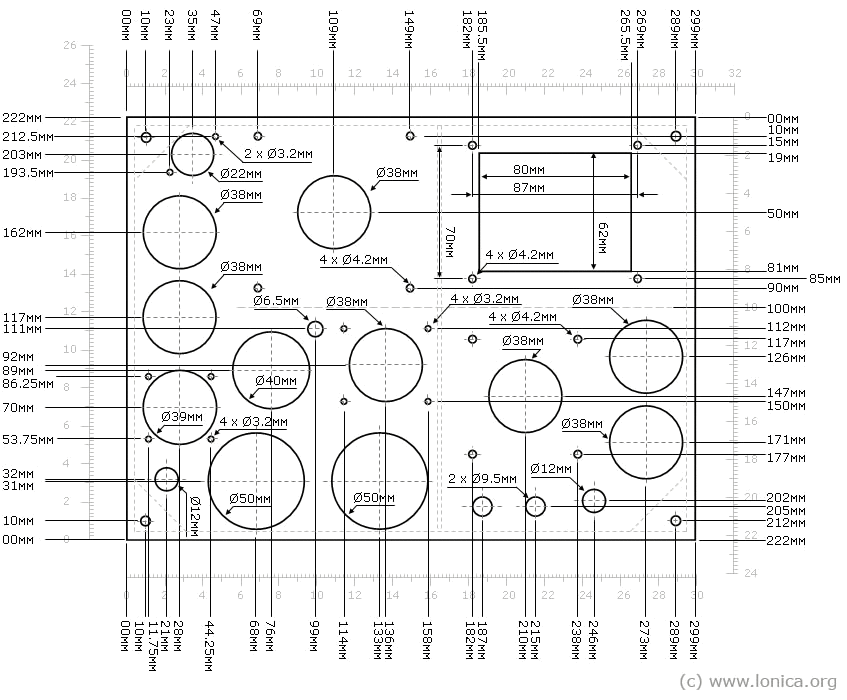Fig. 16. The top mounting panel

### The Bottom Panel. Ventilation Openings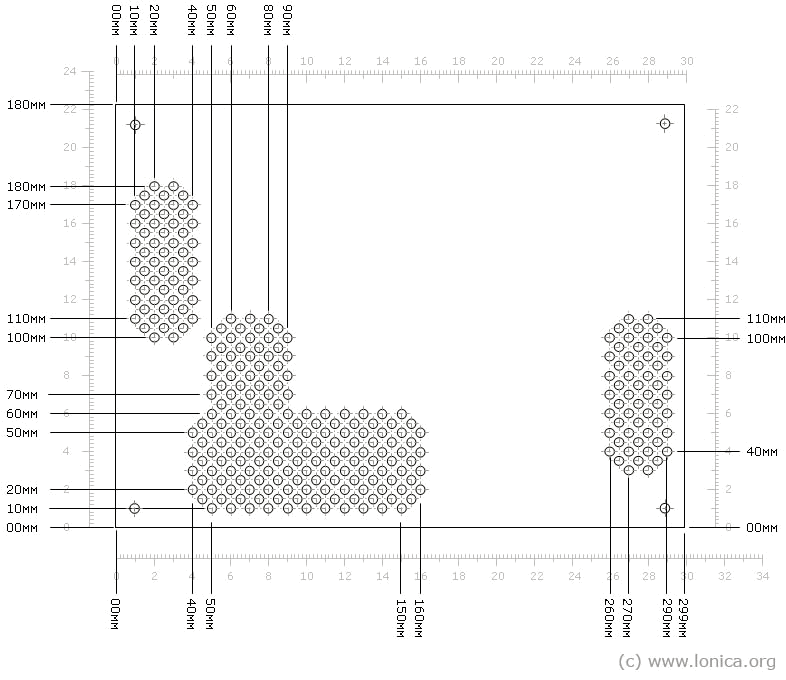Fig. 17. The Bottom Panel. Ventilation Openings

### Arrangement of Parts of the Power Supply. Top ViewFig. 18. Arrangement of Parts of the Power Supply. Top View

### Arrangement of Parts of the Power Supply. Side View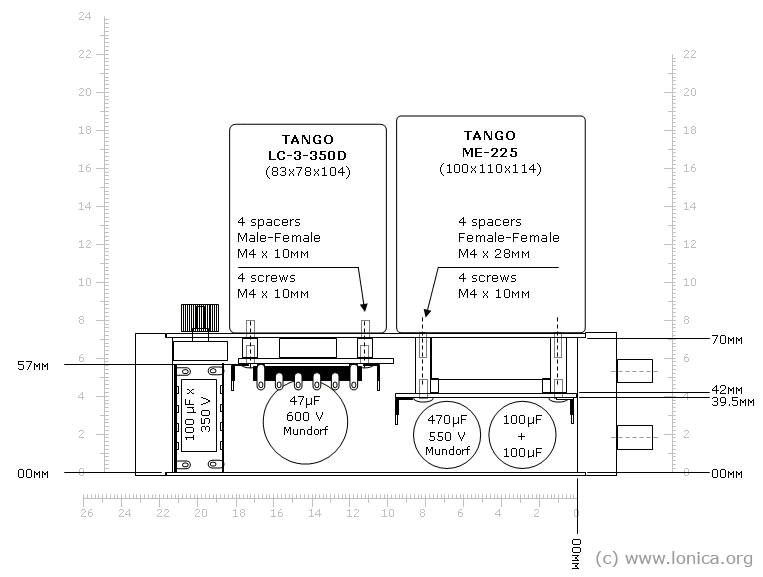Fig. 19. Arrangement of Parts of the Power Supply. Side View

### Internal Mounting Panels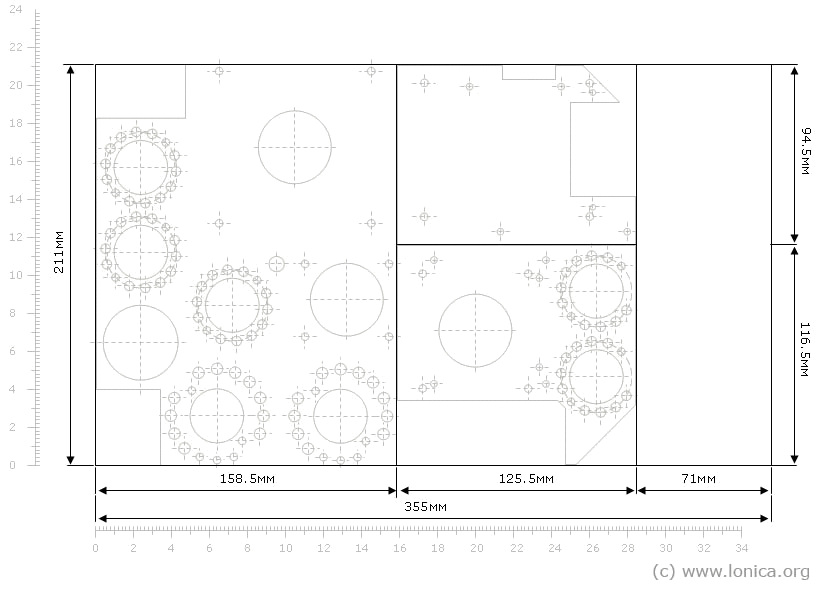Fig. 20. Internal Mounting Panels

### Mounting Panel of the Amplifier Module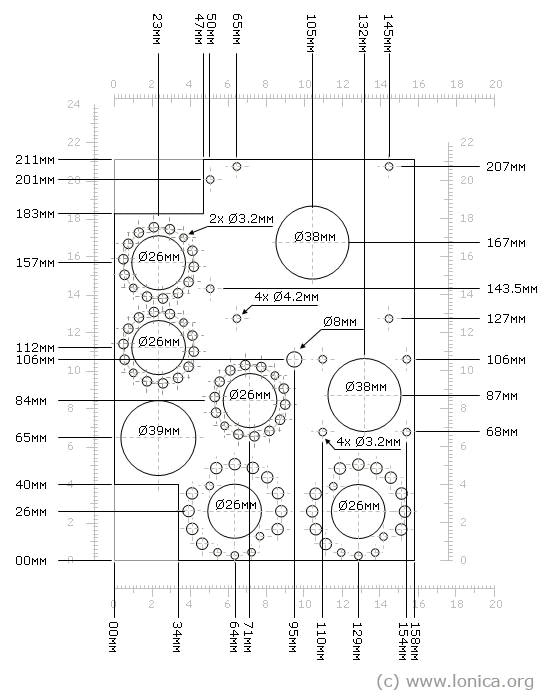Fig. 21. Mounting Panel of the Amplifier Module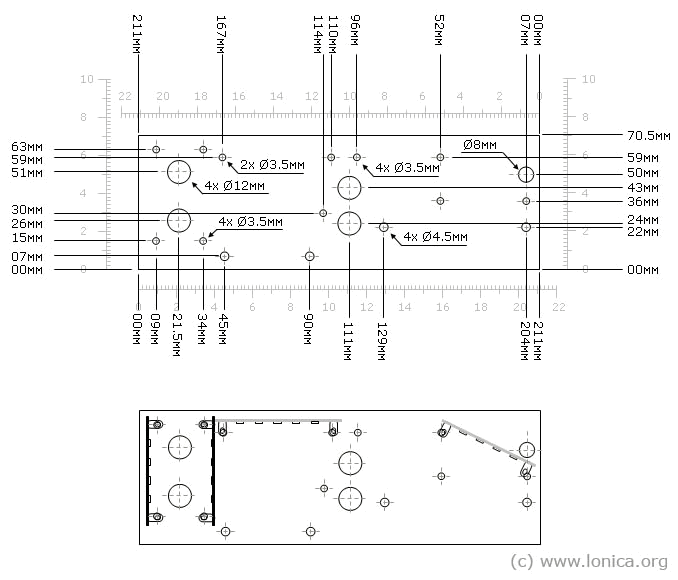### Mounting Panel of the Filament Transformer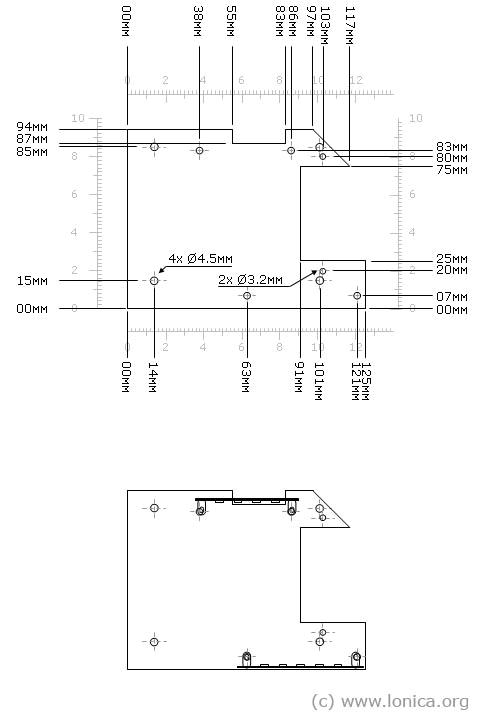Fig. 23. Mounting Panel of the Filament Transformer

### Mounting Panel of the Rectifier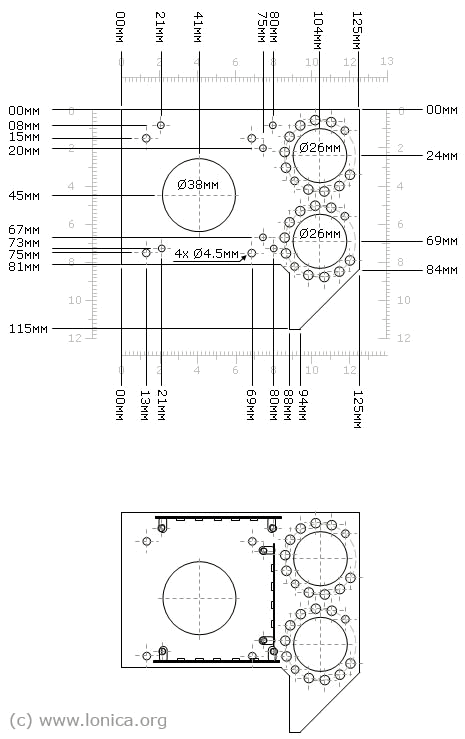Fig. 24. Mounting Panel of the Rectifier

## Gallery

A few images from the different stages of building of the amplifier.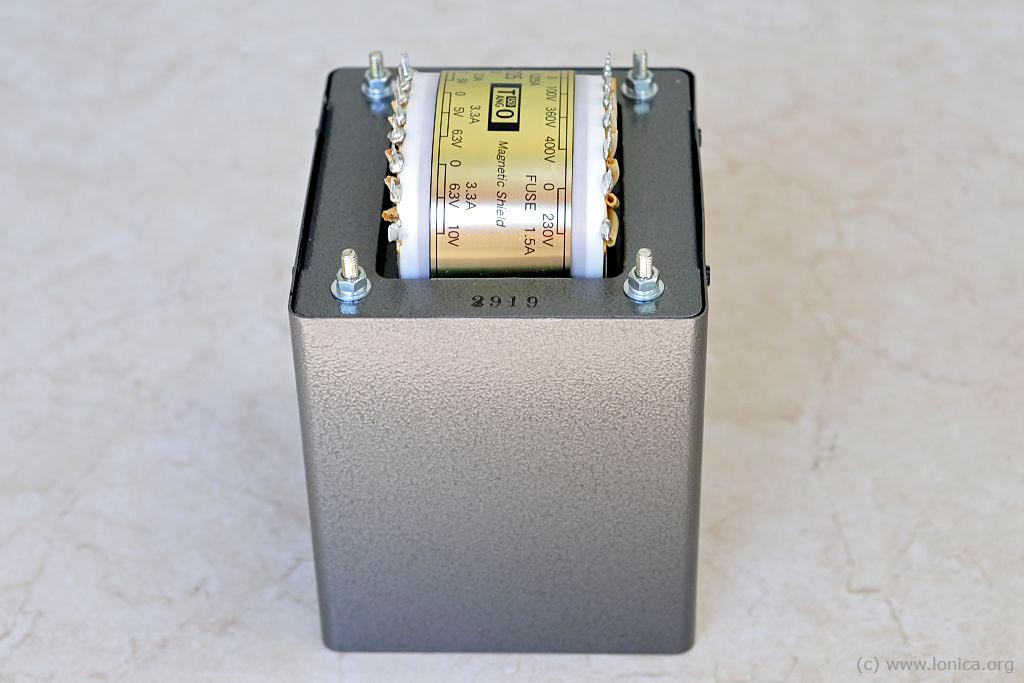Рис. 30. Power Transformer МЕ–225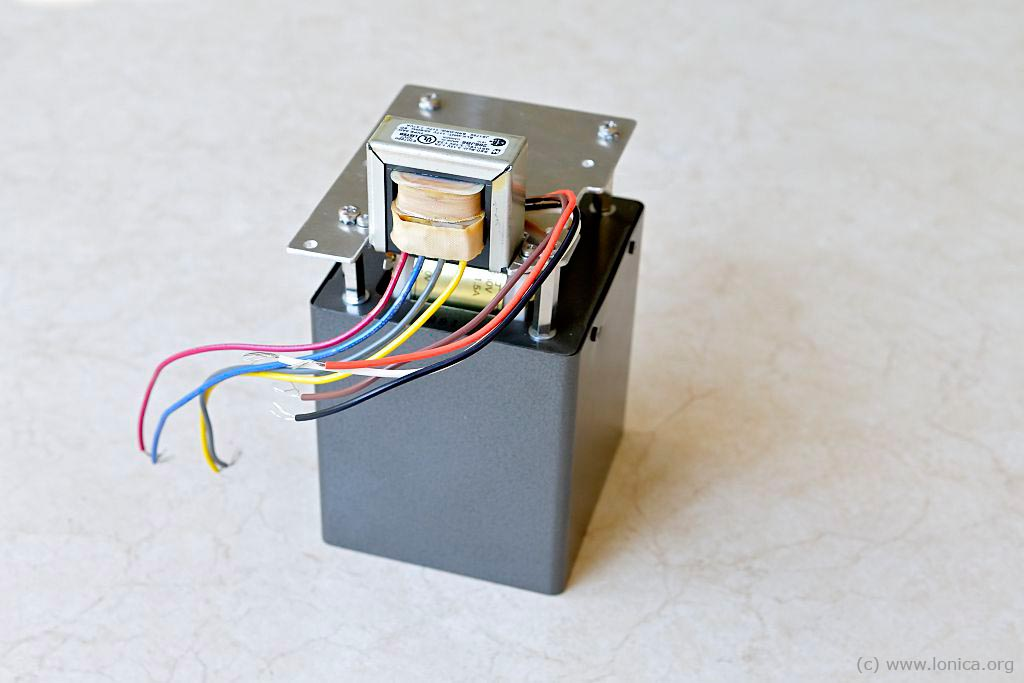Рис. 31. Power Transformer and Filament Transformer on the Mounting Panel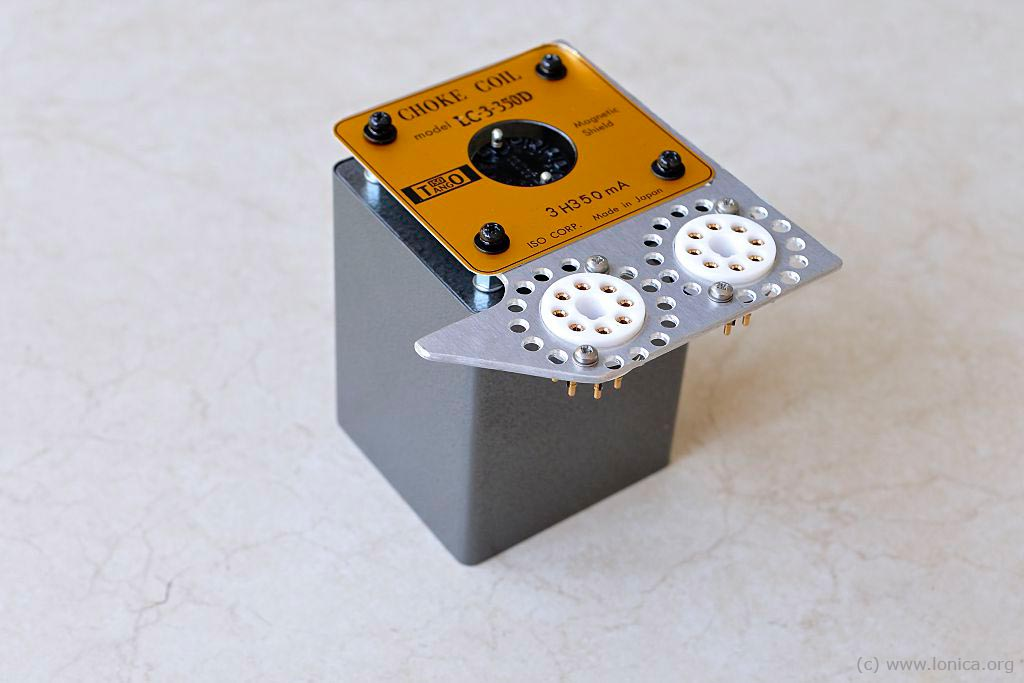Рис. 32. Choke and Sockets of the Rectifiers on the Mounting PanelРис. 33. Routing of the Filament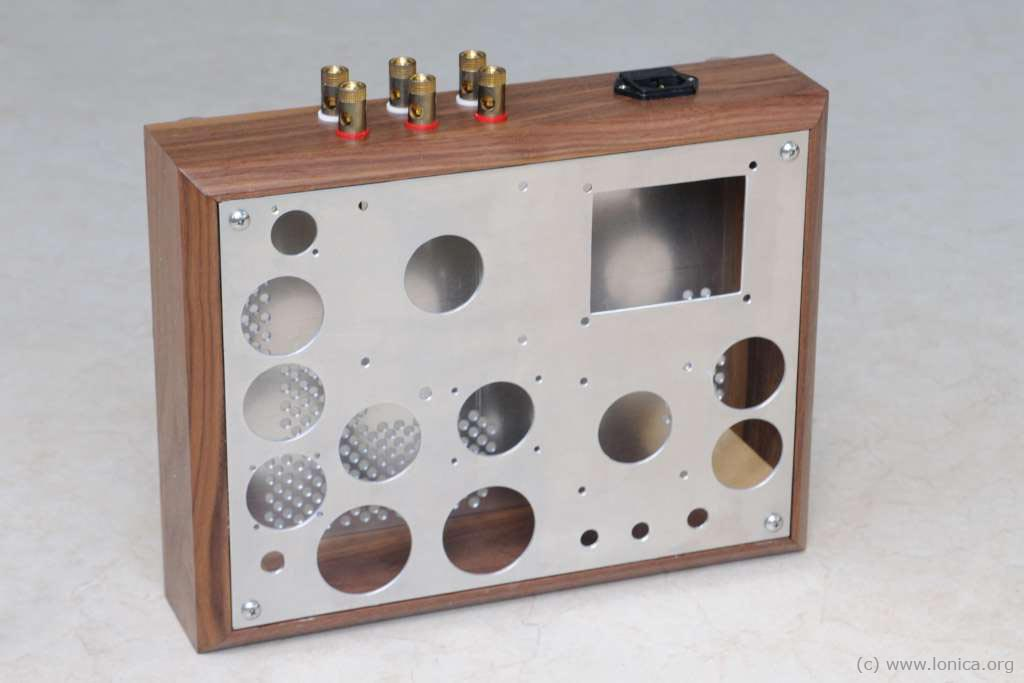Рис. 34. Chassis of the Amplifier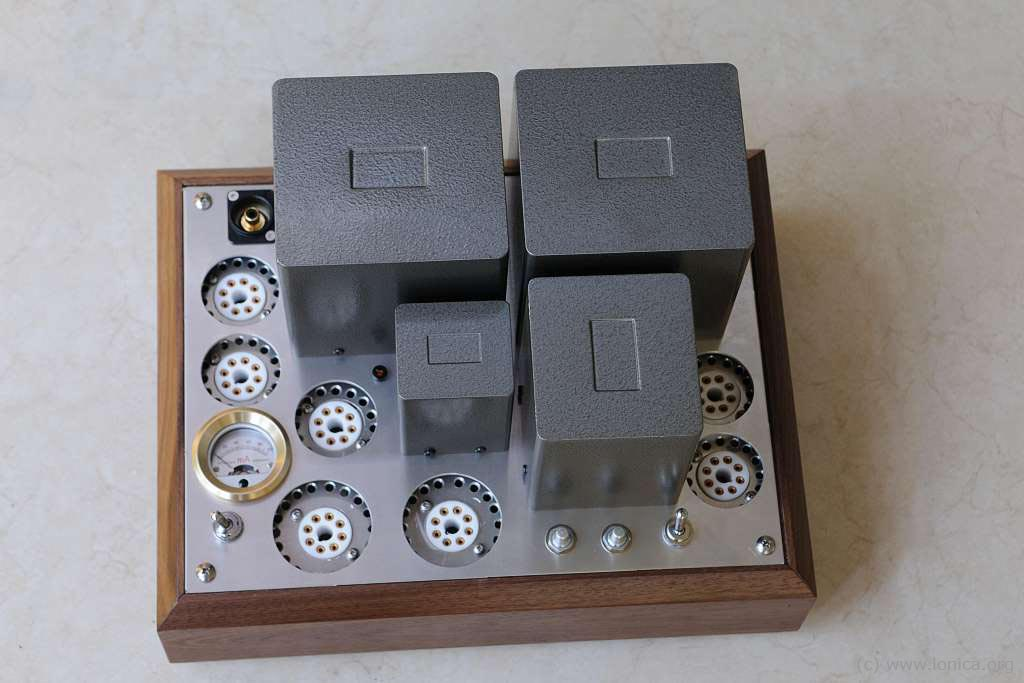Рис. 35. The Amplifier. Top ViewРис. 36. The Amplifier inside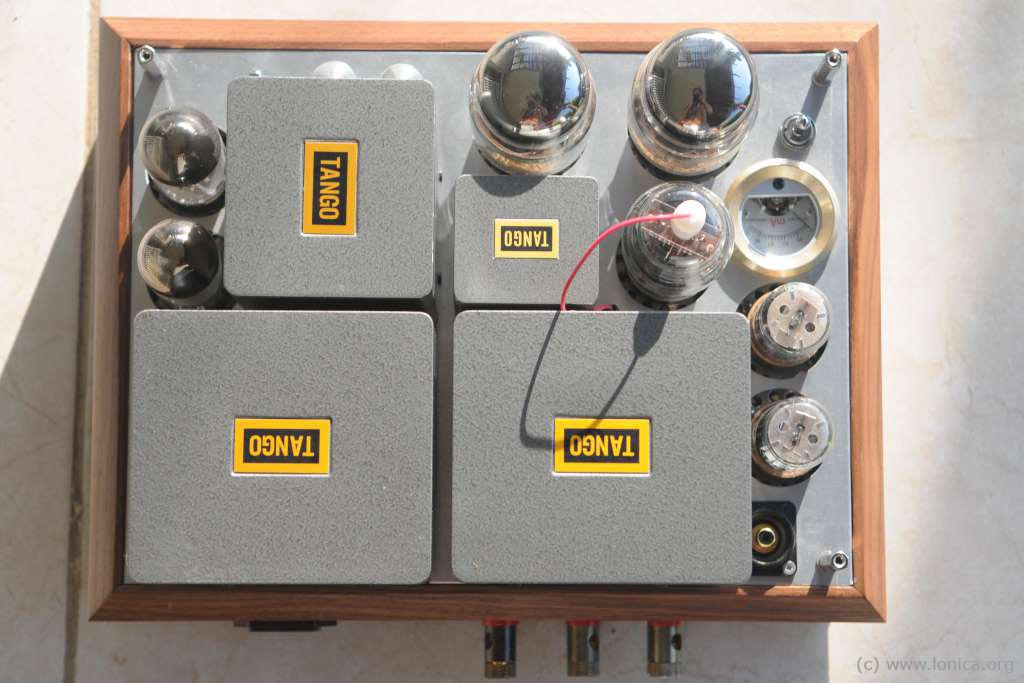Рис. 37. The Amplifier with Tubes. Top View

## Appendix

### The standard range of diameters of drills on metal (mm)

1, 1.5, 2, 2.5, 3, 3.3, 3.5, 4, 4.1, 4.2, 4.5, 5.0, 5.5, 6, 6.5, 7, 7.5, 8, 8.5, 9, 9.5, 10, 10.5, 11, 12, 12.5, 13

### How to read size of screws in inch

For example: #4–40 1/4".

The first digit – number corresponding to the diameter of the screw (diameter = "#" х 0.013" + 0.060").
The second digit – the thread pitch (number of threads per inch): 25.4 / 40 threads = 0.635.
The third digit – length of the screw: 1/4" = 6.35 mm.

Some matching between number of screw and diameter of screw are shown in table below.

№ of screwDiameter (inch)Diameter (mm)
#00.0600"1.5240 mm
#10.0730"1.8542 mm
#20.0860"2.1844 mm
#30.0990"2.5146 mm
#40.1120"2.8448 mm
#50.1250"3.1750 mm
#60.1380" 3.5052 mm
#80.1640"4.1656 mm
#100.1900"4.8260 mm
#120.2160"5.4864 mm

Table 1. Some matching between number of screw and diameter of screw

### Correspondence between U.S. and European diameter of wires

American Wire Gauge (AWG)Diameter (inch)Diameter (mm)Sectional Area (mm2)
00000.4611.68107.16
0000.409610.4084.97
000.36489.2767.40
00.32498.2553.46
10.28937.3542.39
20.25766.5433.61
30.22945.8326.65
40.20435.1921.14
50.18194.6216.76
60.1624.1113.29
70.14433.6710.55
80.12853.268.36
90.11442.916.63
100.10192.595.26
110.09072.304.17
120.08082.053.31
130.0721.832.63
140.06411.632.08
150.05711.451.65
160.05081.291.31
170.04531.151.04
180.04031.020.82
190.03590.910.65
200.0320.810.52
210.02850.720.41
220.02540.650.33
230.02260.570.26
240.02010.510.20
250.01790.450.16
260.01590.400.13

Table 2. Correspondence between U.S. and European diameter of wires

Dimitry S. Lyumet16 March 2014